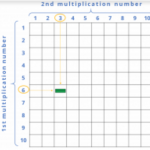Smartick is an advanced online program that teaches kids math and coding in only 15 min. a day

# I want to learn about: Mutiplication

Content about multiplication in elementary mathematics. Exercises, tutorials, problems, and teaching resources for elementary multiplication. Here you will find posts about multiplication to make learning mathematics easier and more fun.

Sep25

## The Associative Property in Addition and Multiplication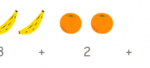Addition and multiplication both use the associative property, while subtraction and division do not. We’re going to to get up close with each situation to get a better idea. The associative property in Addition ♥ Addition indeed has the associative property. Whatever numbers a, b, and c may be, they always end up the same: […]

Sep13

## Understanding Multiplication Problems with Examples

What needs to be done in order to solve multiplication problems? Isn’t it enough to just multiply the numbers that we’re given? No! Because… What’s more important is UNDERSTANDING the multiplication problems. This is why we’re bringing you some multiplication problems today where YOU WON’T NEED TO USE MULTIPLICATION, but rather, TO THINK CRITICALLY. Ready? […]

Sep08

## Review the Different Properties of Multiplication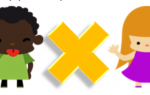Sometimes multiplying can be complicated but knowing the tables and properties of multiplication, we can make this task much easier… let’s get to it! There are three properties of multiplication: commutative, associative, and distributive. Commutative Property It states that the order of the factors does not change the product, that is, it does not matter if […]

Aug28

## Multiplying Numbers Followed by Zeros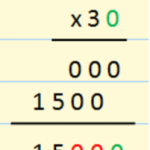In today’s post, we’re going to learn how to multiply numbers that are followed by zeros. You could go about multiplying them like any other number, but we have a trick to teach you, and it’s easy to learn! The most important thing when you learn a trick is to remember why is works the […]

Aug16

## Review Factoring with Examples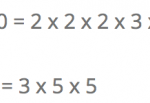Hello again! Do you know what factoring is? Do you know what it is used for? In this post we will answer these questions. How to Factor The factorization of a number is performed by writing the number as the multiplication of all its prime factors. Example: 12 = 2 x 2 x 3 You can […]

Jul31

## Mental Math: Multiplying by 25 and 75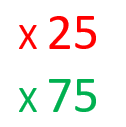In this post we are going to learn a simple way of multiplying by 25 and 75. First, we’ll learn the method and then we’ll give mental math a shot.   Multiplying by 25: The number 25 is 100 divided by 4. So, in order to multiply by 25 what we have to do is […]

Jul21

## Learn the Associative Property of Multiplication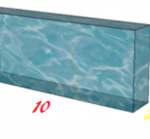The associative property states that the way in which factors are grouped together doesn’t affect the result of an operation: (A x B) x C = A x (B x C) Okay, so we know that the volume of a prism is calculated by multiplying the area of the base by the height: (B x […]

Jul19

## How to Solve a Multiplication ProblemDo you want to know how to solve a multiplication problem? Have you already learned how to multiply? Did you know that solving problems is the best way to learn multiplication? That’s why we are going to look at the different steps that we need to follow in order to solve a multiplication problem. Let’s […]

Jul12

## Learn How to Calculate Factorials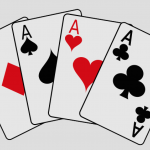Today we are going to learn how to calculate factorials. Calculating factorials is quite simple; let’s see what it’s all about: What is the Factorial Function? We represent the factorial function with the exclamation point “!”, placing it after the number. This exclamation means that we need to multiply all of the whole numbers that […]

Jul05

## Strategy for Multiplying by 2, 4, and 8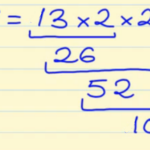In this post, we are going to learn a very simple strategy for multiplying by 2, 4, and 8. Strategy for Multiplying a Number by 2: Multiplying by two is really easy, right? We just have to add the number to itself and we’ll get the answer. For example: 2 x 48 = 48 + 48 […]

May25

## Learn Multiplication Algorithm Using Blocks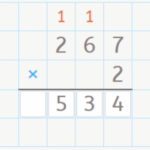Blocks are extremely useful for learning mathematics, and operations in particular. Therefore, this week we’re going to learn the multiplication algorithm using blocks to help us. Do you already know how to multiply by one digit? If the answer is NO, you’ll learn the most simple way to multiply and understand the algorithm in this […]